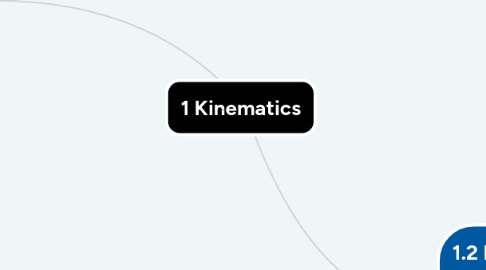# 1 KinematicsJennifer Dick
Get Started. It's Free1 Kinematics## 1. 1.1 Kinematics in One Dimension

### 1.4. 1.1.3 Constant acceleration equations

1.4.1. 1.1.3a Using the constant acceleration equations

1.4.2. 1.1.3b Writing expressions of kinematic quantities

## 2. 1.2 Kinematics in Two Dimensions

### 2.1. 1.2.1a Manipulating displacement and velocity vectors

2.1.1. 1.2.1a1 Determine components of a vector along two specified, mutually perpendicular axes

2.1.2. 1.2.1a2 Determine the net displacement of a particle or the location of a particle relative to another

2.1.3. 1.2.1a3 Determine the change in velocity of a particle or the velocity of one particle relative to another

### 2.5. 1.2.3 Understanding uniform circular motion

2.5.1. 1.2.3a Relate the radius of the circle and the speed or rate of revolution of the particle to the magnitude of the centripetal acceleration.

2.5.2. 1.2.3b Describe the direction of the particle’s velocity and acceleration at any instant during the motion.

2.5.3. 1.2.3c Determine the components of the velocity and acceleration vectors at any instant, and sketch or identify graphs of these quantities.

### 2.6. 1.2.4 Understanding frames of reference

2.6.1. 1.2.4a Analyze the uniform motion of an object relative to a moving medium such as a flowing stream.

2.6.2. 1.2.4b Analyze the motion of particles relative to a frame of reference that is accelerating horizontally or vertically at a uniform rate.